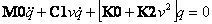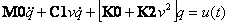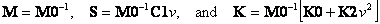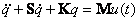Applying Control Theory to Bicycle Linearized Equations of Motion

Applying Control Theory to Bicycle Linearized Equations of Motion

The Biorobotics and Locomotion Laboratory

Department of Theoretical and Applied Mechanics

Cornell University, Ithaca, NY

2003-2015

Abstract

It is possible to plug the linearized equations of motion for an idealized bicycle, contained in JBike6, into control algorithms. Below is an example of that in MATLAB.Contents

Introduction

# Introduction

In order to understand what is required for a rider to control a bicycle, it may be useful to apply control theory. One requirement is the linearized equations of motion, which JBike6 can provide. Below is an example of using the equations of motion from JBike6 to create a transfer function which can be analyzed with MATLAB’s  Control System Toolbox. The example goes so far as to create a pole-zero map which exactly matches the plot of eigenvalues generated by JBike6.

# Linearized equations of motion for an uncontrolled bicycle

M0*qdd+(C1.*v)*qd+(K0+K2.*v^2)*q=0

i.ewherev = forward speed

M0 = Mass matrix

C1 = Velocity sensitivity matrix

K0 and K2 = Stiffness matrices

# Define Input and Output

Let the control 'input' u(t) be steer torque τ and the 'output' y(t) be lean angle. ThenMultiply through by M0-1 now, to avoid inverting a singular matrix laterFor computational simplicity, let, so# Represent in state-space form, and, recast from 2 coupled 2nd-order equations to 4 1st-order equations.

To that end, let,,, and. Thusbecomes,

which can be written asorSo, finally,,, and# Create Transfer Function

We can convert the above to the numerator and denominator of a transfer function with MATLAB's ss2tf() function

[numG, denG] = ss2tf(A,B,C,D)

Then we can combine them into a single transfer function with MATLAB's tf() function

G = tf(numG, denG)

# Plot Poles

We can then use MATLAB’s pole-zero map function: [p, z] = pzmap(G)

Which, for the Schwinn Crown, has poles that look like this: a perfect match for the eigenvalues generated in JBike6# The MATLAB source code

% From JBike6

% The linearized equations of motion read:

%

%       M0*qdd+(C1.*v)*qd+(K0+K2.*v^2)*q=0

%

% where v is the forward speed of the bike.

%

% Requires MATLAB’s Control System Toolbox

% set the bicycle parameters

JBini

% calculate the linearized equations of motion

JBmck

% calculate the weave and capsize speed

JBvcrit

% calculate the eigenvalues and eigenvectors for a speed range

JBeig

% plot only the Real part of the eigenvalues

subplot(1,2,1);

JBrev

title('JBike6'); a = axis;

drawnow;

% create transfer function from state-space representation

% use x1 = q1, x2 = q1_dot, x3 = q2, x4 = q2_dot

subplot(1,2,2);

v = 0:0.1:10;

M = inv(M0);

for i = 1: length(v)

S = M*(C1.*v(i));

K = M*(K0 + K2.*v(i)^2);

A = -[0,      -1,     0,      0;

K(1,1), S(1,1), K(1,2), S(1,2);

0,      0,      0,      -1;

K(2,1), S(2,1), K(2,2), S(2,2)];

B = [0, 0,      0, 0;

0, M(1,1), 0, M(1,2);

0, 0,      0, 0;

0, M(2,1), 0, M(2,2)];

C = [1, 0, 0, 0];

D = [0, 0, 0, 0];

[numG, denG] = ss2tf(A, B, C, D, 2);

G = tf(numG, denG);

[p, z] = pzmap(G);

plot(v(i).*ones(1, length(p)), p, '.'); hold on;

%rlocus(G)

%sisotool(G);

end

grid on; hold off; axis(a);

title('Poles of Transfer Function');

 JBike6 Source Code, Created by Arend L. Schwab and Jim Papadopoulos, 18-Jun-2003